# Performance of Sparse Recovery Using L1 Minimization¶

Important: Please read the installation page for details about how to install the toolboxes. $\newcommand{\dotp}{\langle #1, #2 \rangle}$ $\newcommand{\enscond}{\lbrace #1, #2 \rbrace}$ $\newcommand{\pd}{ \frac{ \partial #1}{\partial #2} }$ $\newcommand{\umin}{\underset{#1}{\min}\;}$ $\newcommand{\umax}{\underset{#1}{\max}\;}$ $\newcommand{\umin}{\underset{#1}{\min}\;}$ $\newcommand{\uargmin}{\underset{#1}{argmin}\;}$ $\newcommand{\norm}{\|#1\|}$ $\newcommand{\abs}{\left|#1\right|}$ $\newcommand{\choice}{ \left\{ \begin{array}{l} #1 \end{array} \right. }$ $\newcommand{\pa}{\left(#1\right)}$ $\newcommand{\diag}{{diag}\left( #1 \right)}$ $\newcommand{\qandq}{\quad\text{and}\quad}$ $\newcommand{\qwhereq}{\quad\text{where}\quad}$ $\newcommand{\qifq}{ \quad \text{if} \quad }$ $\newcommand{\qarrq}{ \quad \Longrightarrow \quad }$ $\newcommand{\ZZ}{\mathbb{Z}}$ $\newcommand{\CC}{\mathbb{C}}$ $\newcommand{\RR}{\mathbb{R}}$ $\newcommand{\EE}{\mathbb{E}}$ $\newcommand{\Zz}{\mathcal{Z}}$ $\newcommand{\Ww}{\mathcal{W}}$ $\newcommand{\Vv}{\mathcal{V}}$ $\newcommand{\Nn}{\mathcal{N}}$ $\newcommand{\NN}{\mathcal{N}}$ $\newcommand{\Hh}{\mathcal{H}}$ $\newcommand{\Bb}{\mathcal{B}}$ $\newcommand{\Ee}{\mathcal{E}}$ $\newcommand{\Cc}{\mathcal{C}}$ $\newcommand{\Gg}{\mathcal{G}}$ $\newcommand{\Ss}{\mathcal{S}}$ $\newcommand{\Pp}{\mathcal{P}}$ $\newcommand{\Ff}{\mathcal{F}}$ $\newcommand{\Xx}{\mathcal{X}}$ $\newcommand{\Mm}{\mathcal{M}}$ $\newcommand{\Ii}{\mathcal{I}}$ $\newcommand{\Dd}{\mathcal{D}}$ $\newcommand{\Ll}{\mathcal{L}}$ $\newcommand{\Tt}{\mathcal{T}}$ $\newcommand{\si}{\sigma}$ $\newcommand{\al}{\alpha}$ $\newcommand{\la}{\lambda}$ $\newcommand{\ga}{\gamma}$ $\newcommand{\Ga}{\Gamma}$ $\newcommand{\La}{\Lambda}$ $\newcommand{\si}{\sigma}$ $\newcommand{\Si}{\Sigma}$ $\newcommand{\be}{\beta}$ $\newcommand{\de}{\delta}$ $\newcommand{\De}{\Delta}$ $\newcommand{\phi}{\varphi}$ $\newcommand{\th}{\theta}$ $\newcommand{\om}{\omega}$ $\newcommand{\Om}{\Omega}$

This tour explores theoritical garantees for the performance of recovery using $\ell^1$ minimization.

In :
options(warn=-1) # turns off warnings, to turn on: "options(warn=0)"
library(pracma)
library(imager)

# Importing the libraries
for (f in list.files(path="nt_toolbox/toolbox_general/", pattern="*.R")) {
source(paste("nt_toolbox/toolbox_general/", f, sep=""))
}
for (f in list.files(path="nt_toolbox/toolbox_signal/", pattern="*.R")) {
source(paste("nt_toolbox/toolbox_signal/", f, sep=""))
}


## Sparse $\ell^1$ Recovery¶

We consider the inverse problem of estimating an unknown signal $x_0 \in \RR^N$ from noisy measurements $y=\Phi x_0 + w \in \RR^P$ where $\Phi \in \RR^{P \times N}$ is a measurement matrix with $P \leq N$, and $w$ is some noise.

This tour is focused on recovery using $\ell^1$ minimization $$x^\star \in \uargmin{x \in \RR^N} \frac{1}{2}\norm{y-\Phi x}^2 + \la \norm{x}_1.$$

Where there is no noise, we consider the problem $\Pp(y)$ $$x^\star \in \uargmin{\Phi x = y} \norm{x}_1.$$

We are not concerned here about the actual way to solve this convex problem (see the other numerical tours on sparse regularization) but rather on the theoritical analysis of wether $x^\star$ is close to $x_0$.

More precisely, we consider the following three key properties

• Noiseless identifiability: $x_0$ is the unique solution of $\Pp(y)$ for $y=\Phi x_0$.
• Robustess to small noise: one has $\norm{x^\star - x_0} = O(\norm{w})$ for $y=\Phi x_0+w$ if $\norm{w}$ is smaller than an arbitrary small constant that depends on $x_0$ if $\la$ is well chosen according to $\norm{w}$.
• Robustess to bounded noise: same as above, but $\norm{w}$ can be arbitrary.

Note that noise robustness implies identifiability, but the converse is not true in general.

## Coherence Criteria¶

The simplest criteria for identifiality are based on the coherence of the matrix $\Phi$ and depends only on the sparsity $\norm{x_0}_0$ of the original signal. This criteria is thus not very precise and gives very pessimistic bounds.

The coherence of the matrix $\Phi = ( \phi_i )_{i=1}^N \in \RR^{P \times N}$ with unit norm colum $\norm{\phi_i}=1$ is $$\mu(\Phi) = \umax{i \neq j} \abs{\dotp{\phi_i}{\phi_j}}.$$

Compute the correlation matrix (remove the diagonal of 1's).

In :
remove_diag = function(C){C - base::diag(base::diag(C))}
Correlation = function(Phi){remove_diag(abs(t(Phi) %*% Phi))}


Compute the coherence $\mu(\Phi)$.

In :
mu = function(Phi){max(Correlation(Phi))}


The condition $$\norm{x_0}_0 < \frac{1}{2}\pa{1 + \frac{1}{\mu(\Phi)}}$$ implies that $x_0$ is identifiable, and also implies to robustess to small and bounded noise.

Equivalently, this condition can be written as $\text{Coh}(\norm{x_0}_0)<1$ where $$\text{Coh}(k) = \frac{k \mu(\Phi)}{ 1 - (k-1)\mu(\Phi) }$$

In :
Coh = function(Phi, k){(k * mu(Phi)) / (1 - (k-1) * mu(Phi))}


Generate a matrix with random unit columns in $\RR^P$.

In :
normalize = function(Phi){t(t(Phi) / sqrt(apply(Phi**2,2, sum)))}
PhiRand = function(P, N){normalize(matrix( rnorm(P*N,mean=0,sd=1), P, N))}
Phi = PhiRand(250, 1000)


Compute the coherence and the maximum possible sparsity to ensure recovery using the coherence bound.

In :
c = mu(Phi)
print(paste("Coherence:", round(c, 2)))
print(paste("Sparsity max:", floor(1/2*(1 + 1/c))))

 "Coherence: 0.32"
 "Sparsity max: 2"


Exercise 1

Display how the average coherence of a random matrix decays with the redundancy $\eta = P/N$ of the matrix $\Phi$. Can you derive an empirical law between $P$ and the maximal sparsity?

In :
source("nt_solutions/sparsity_6_l1_recovery/exo1.R")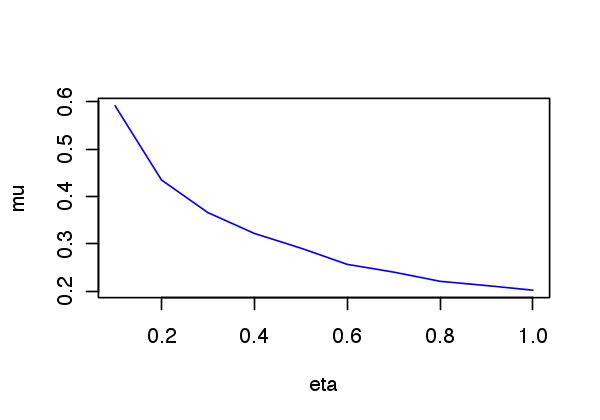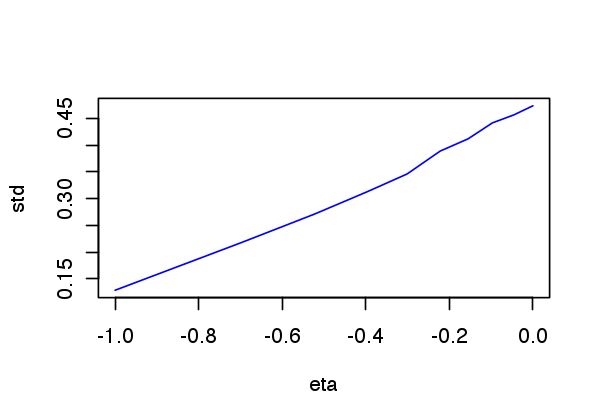In :
##Insert your code here.


## Support and Sign-based Criteria¶

In the following we will consider the support $$\text{supp}(x_0) = \enscond{i}{x_0(i) \neq 0}$$ of the vector $x_0$. The co-support is its complementary $I^c$.

In :
where = function(I)
{
n = length(I)
out = c()
for (i in 1:n)
{
if (I[i] == TRUE)
{
out = c(out, i)
}
}
return(out)
}

supp   = function(s){where(abs(s) > 1e-5)}
cosupp = function(s){where(abs(s) < 1e-5)}


Given some support $I \subset \{0,\ldots,N-1\}$, we will denote as $\Phi = (\phi_m)_{m \in I} \in \RR^{N \times \abs{I}}$ the sub-matrix extracted from $\Phi$ using the columns indexed by $I$.

J.J. Fuchs introduces a criteria $F$ for identifiability that depends on the sign of $x_0$.

J.J. Fuchs. Recovery of exact sparse representations in the presence of bounded noise. IEEE Trans. Inform. Theory, 51(10), p. 3601-3608, 2005

Under the condition that $\Phi_I$ has full rank, the $F$ measure of a sign vector $s \in \{+1,0,-1\}^N$ with $\text{supp}(s)=I$ reads $$\text{F}(s) = \norm{ \Psi_I s_I }_\infty \qwhereq \Psi_I = \Phi_{I^c}^* \Phi_I^{+,*}$$ where $A^+ = (A^* A)^{-1} A^*$ is the pseudo inverse of a matrix $A$.

The condition $$\text{F}(\text{sign}(x_0))<1$$ implies that $x_0$ is identifiable, and also implies to robustess to small noise. It does not however imply robustess to a bounded noise.

Compute $\Psi_I$ matrix.

In :
PsiI = function(Phi,I)
{
keep_indices = c()
for (i in 1:dim(Phi))
{
if (!(i %in% I))
{
keep_indices = c(keep_indices, i)
}
}
return (t(Phi[,keep_indices]) %*% t(pinv(as.matrix(Phi[,I]))))
}


Compute $\text{F}(s)$.

In :
F = function(Phi,s){base::norm(PsiI(Phi, supp(s)) %*% s[supp(s)], type="I")}


The Exact Recovery Criterion (ERC) of a support $I$, introduced by Tropp in

J. A. Tropp. Just relax: Convex programming methods for identifying sparse signals. IEEE Trans. Inform. Theory, vol. 52, num. 3, pp. 1030-1051, Mar. 2006.

Under the condition that $\Phi_I$ has full rank, this condition reads $$\text{ERC}(I) = \norm{\Psi_{I}}_{\infty,\infty} = \umax{j \in I^c} \norm{ \Phi_I^+ \phi_j }_1.$$ where $\norm{A}_{\infty,\infty}$ is the $\ell^\infty-\ell^\infty$ operator norm of a matrix $A$.

In :
erc = function(Phi, I){base::norm(PsiI(Phi, I), type="I")}


The condition $$\text{ERC}(\text{supp}(x_0))<1$$ implies that $x_0$ is identifiable, and also implies to robustess to small and bounded noise.

One can prove that the ERC is the maximum of the F criterion for all signs of the given support $$\text{ERC}(I) = \umax{ s, \text{supp}(s) \subset I } \text{F}(s).$$

The weak-ERC is an approximation of the ERC using only the correlation matrix $$\text{w-ERC}(I) = \frac{ \umax{j \in I^c} \sum_{i \in I} \abs{\dotp{\phi_i}{\phi_j}} }{ 1-\umax{j \in I} \sum_{i \neq j \in I} \abs{\dotp{\phi_i}{\phi_j}} }$$

In :
setdiff = function(n, I)
{keep_indices = c()
for (i in 1:n)
{
if (!(i %in% I))
{
keep_indices = c(keep_indices, i)
}
}
return(keep_indices)
}

g = function(C,I){apply(as.matrix(C[,I]), 1, sum)}
werc_g = function(g,I,J){max(g[J])/(1 - max(g[I]))}
werc = function(Phi,I){werc_g(g(Correlation(Phi), I), I, setdiff(dim(Phi), I))}


One has, if $\text{w-ERC}(I)>0$, for $I = \text{supp}(s)$, $$\text{F}(s) \leq \text{ERC}(I) \leq \text{w-ERC}(I) \leq \text{Coh}(\abs{I}).$$

This shows in particular that the condition $$\text{w-ERC}(\text{supp}(x_0))<1$$ implies identifiability and robustess to small and bounded noise.

Exercise 2

Show that this inequality holds on a given matrix. What can you conclude about the sharpness of these criteria ?

In :
source("nt_solutions/sparsity_6_l1_recovery/exo2.R")

 "N = 2000 , P = 1990 , |I| = 6"
 "F(s) = 0.19"
 "ERC(I) = 0.23"
 "w-ERC(s) = 0.27"
 "Coh(|s|) = 1.65"

In :
## Insert your code here.


Exercise 3

For a given matrix $\Phi$ generated using PhiRand, draw as a function of the sparsity $k$ the probability that a random sign vector $s$ of sparsity $\norm{s}_0=k$ satisfies the conditions $\text{F}(x_0)<1$, $\text{ERC}(x_0)<1$ and $\text{w-ERC}(x_0)<1$

In :
source("nt_solutions/sparsity_6_l1_recovery/exo3.R")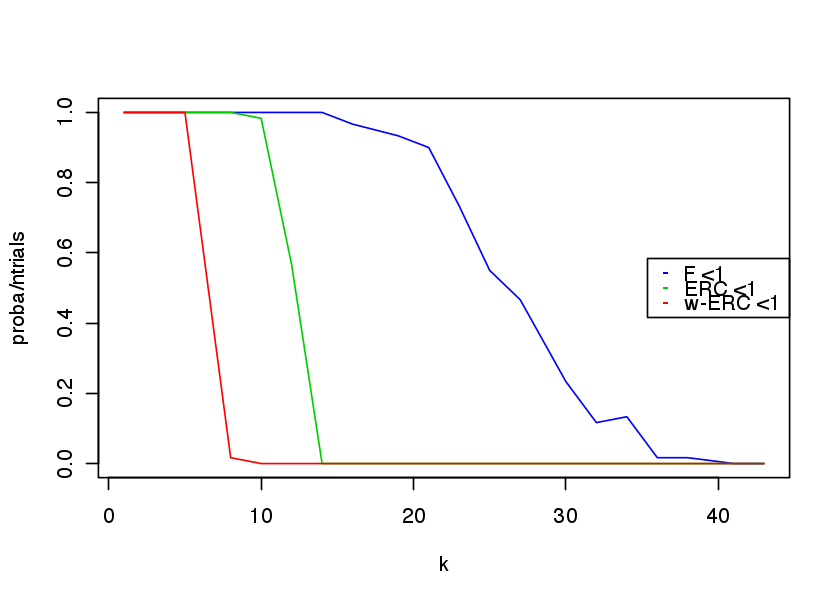In :
#Insert your code here.


## Restricted Isometry Criteria¶

The restricted isometry constants $\de_k^1,\de_k^2$ of a matrix $\Phi$ are the smallest $\de^1,\de^2$ that satisfy $$\forall x \in \RR^N, \quad \norm{x}_0 \leq k \qarrq (1-\de^1)\norm{x}^2 \leq \norm{\Phi x}^2 \leq (1+\de^2)\norm{x}^2.$$

E. Candes shows in

E. J. Cand s. The restricted isometry property and its implications for compressed sensing. Compte Rendus de l'Academie des Sciences, Paris, Serie I, 346 589-592

that if $$\de_{2k} \leq \sqrt{2}-1 ,$$ then $\norm{x_0} \leq k$ implies identifiability as well as robustness to small and bounded noise.

The stability constant $\la^1(A), \la^2(A)$ of a matrix $A = \Phi_I$ extracted from $\Phi$ is the smallest $\tilde \la_1,\tilde \la_2$ such that $$\forall \al \in \RR^{\abs{I}}, \quad (1-\tilde\la_1)\norm{\al}^2 \leq \norm{A \al}^2 \leq (1+\tilde \la_2)\norm{\al}^2.$$

These constants $\la^1(A), \la^2(A)$ are easily computed from the largest and smallest eigenvalues of $A^* A \in \RR^{\abs{I} \times \abs{I}}$

In :
minmax = function(v){c(1 - min(v), max(v) - 1)}
ric = function(A){minmax(eigen(t(A) %*% A)$values)}  The restricted isometry constant of$\Phi$are computed as the largest stability constants of extracted matrices $$\de^\ell_k = \umax{ \abs{I}=k } \la^\ell( \Phi_I ).$$ The eigenvalues of$\Phi$are essentially contained in the interval$ [a,b] $where$a=(1-\sqrt{\be})^2$and$b=(1+\sqrt{\be})^2$with$\beta = k/P$More precisely, as$k=\be P$tends to infinity, the distribution of the eigenvalues tends to the Marcenko-Pastur law$ f_\be(\la) = \frac{1}{2\pi \be \la}\sqrt{ (\la-b)^+ (a-\la)^+ }. $Exercise 4 Display, for an increasing value of$k$the histogram of repartition of the eigenvalues$A^* A$where$A$is a Gaussian matrix of size$(P,k)$and variance$1/P$. For this, accumulate the eigenvalues for many realizations of$A$. In : source("nt_solutions/sparsity_6_l1_recovery/exo4.R")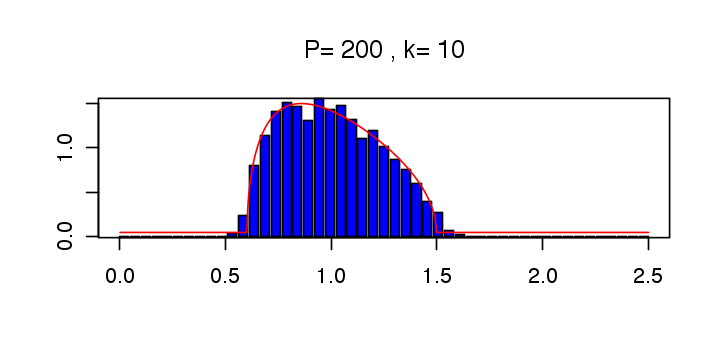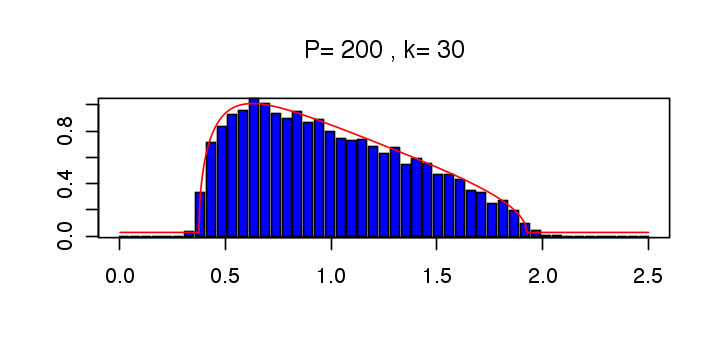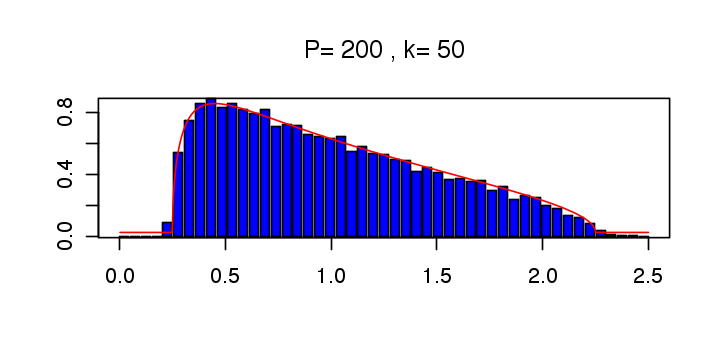In : ## Insert your code here.  Exercise 5 Estimate numerically lower bound on$\de_k^1,\de_k^2$by Monte-Carlo sampling of sub-matrices. In : source("nt_solutions/sparsity_6_l1_recovery/exo5.R")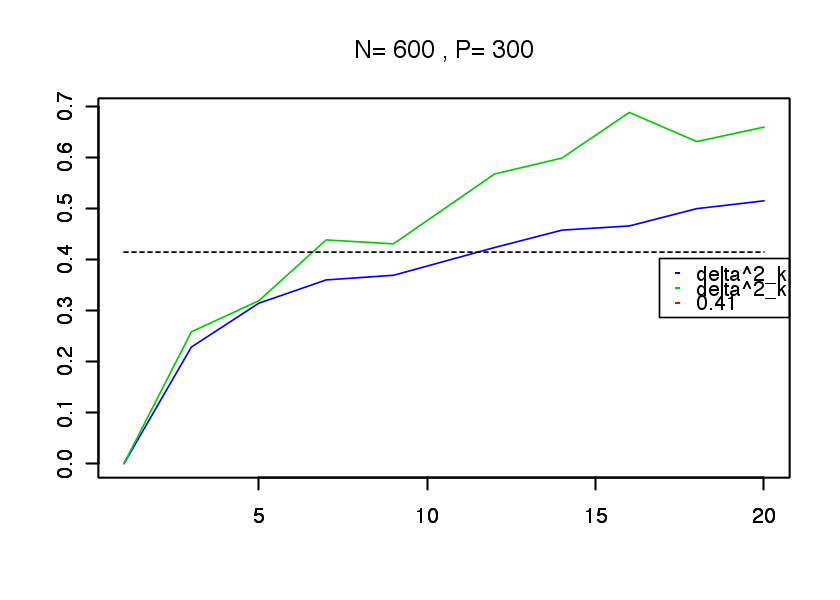In : ## Insert your code here.  ## Sparse Spikes Deconvolution¶ We now consider a convolution dictionary$ \Phi $. Such a dictionary is used with sparse regulariz Second derivative of Gaussian kernel$g$with a given variance$\si^2$. In : sigma = 6 g = function(x){(1 - (x**2 / sigma**2)) * exp(-x**2/(2*sigma**2))}  Create a matrix$\Phi$so that$\Phi x = g \star x$with periodic boundary conditions. In : P = 1024 t = meshgrid(0:(P - 1),0:(P - 1)) Y = t$X
X = t$Y Phi = normalize(g((X - Y + P/2.) %% P - P/2.))  To improve the conditionning of the dictionary, we sub-sample its atoms, so that$ P = \eta N > N $. In : eta = 2. N = P/eta Phi = Phi[,seq(1, dim(Phi), by=eta)]  Plot the correlation function associated to the filter. Can you determine the value of the coherence$\mu(\Phi)$? In : c = t(Phi) %*% Phi c = abs(c[,dim(c)/2]) options(repr.plot.width=5, repr.plot.height=5) plot(c[(length(c)/2 - 50):((length(c)/2) + 50)], type="l", xlab="", ylab="", col=4)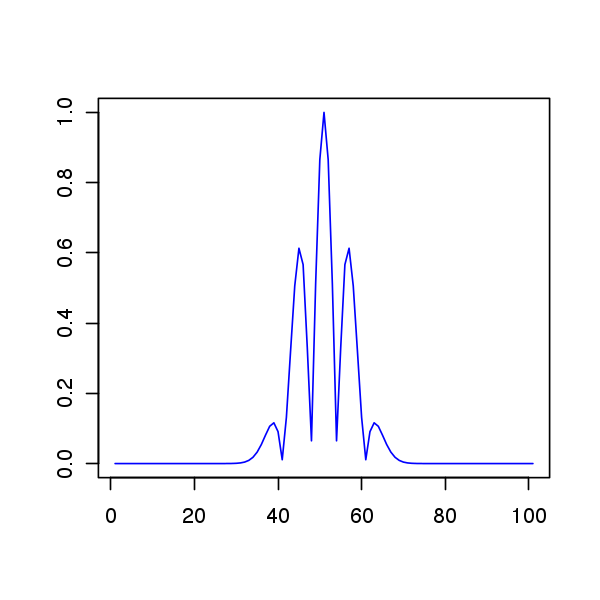In : roll <- function( x , n ){ if( n == 0 ) return( x ) c( tail(x,n) , head(x,-n) ) }  In : twosparse = function(d){roll(c(1, rep(0, d), -1, rep(0, N - d- 2)), as.integer(N/2 - d/2))}  Display$x_0$and$\Phi x_0$. In : x0 = twosparse(50) plot(x0, type="l", col=2, ylab="", xlab="") plot(Phi %*% x0, type="l", col=4, ylab="", xlab="")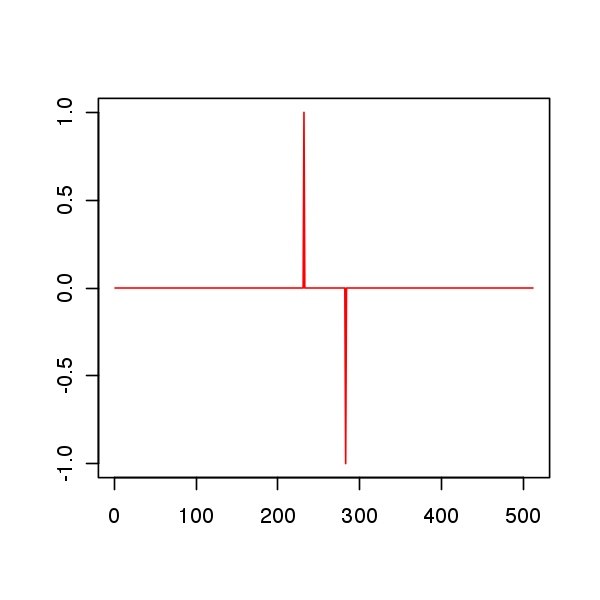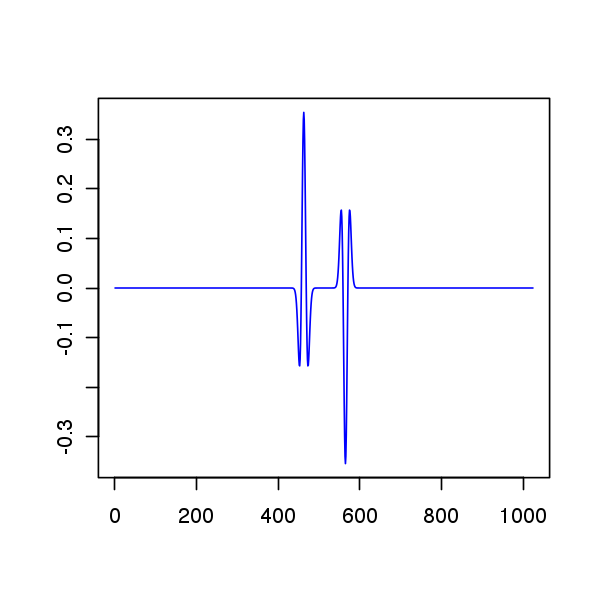Exercise 6 Plot the evolution of the criteria F, ERC and Coh as a function of$d$. Do the same plot for other signs patterns for$x_0$. Do the same plot for a Dirac comb with a varying spacing$d\$.

In :
source("nt_solutions/sparsity_6_l1_recovery/exo6.R")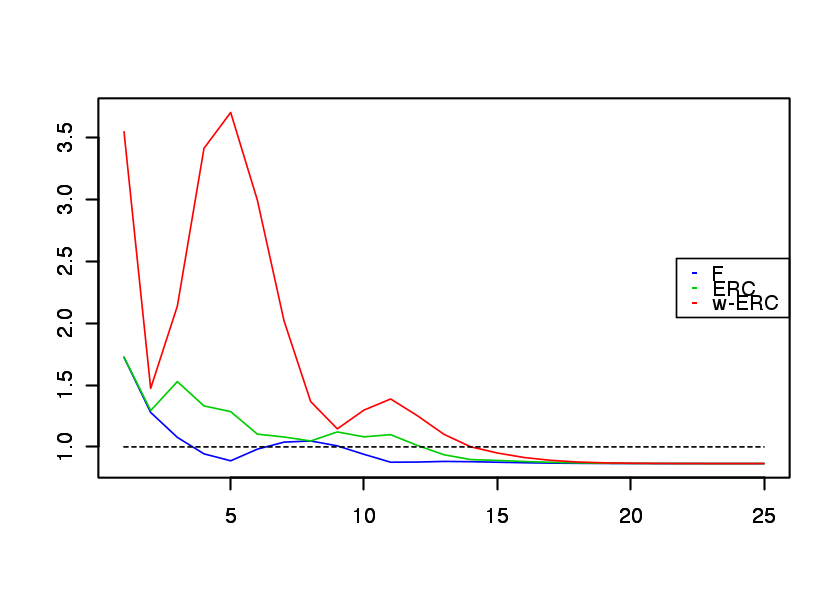In :
#Insert your code here.